# Digging Deep

R
time series
correlation
apps
tables
textual analysis
Do we see more planning applications when house sales are depressed?
Author

Carl Goodwin

Published

January 10, 2018

Modified

February 24, 2023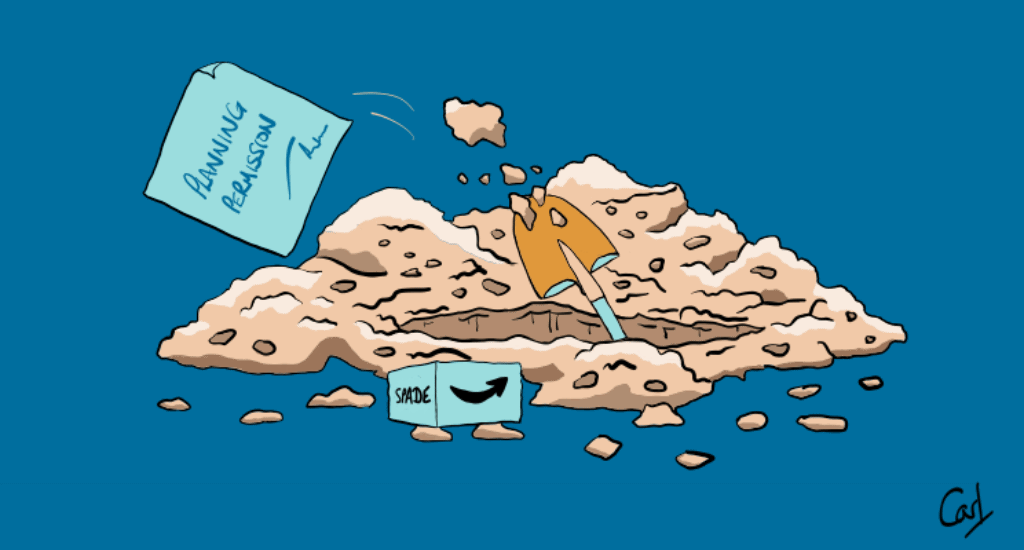In House Sales I looked at how a series of events damped down sales. By combining these sales data with planning applications I’d like to see if home owners “start digging” when they can’t sell.

Planning data is harvested with the kind permission of The Royal Borough of Kensington and Chelsea (RBKC). The code for these code chunks is not rendered out of courtesy to RBKC.

``````library(conflicted)
library(tidyverse)
conflict_prefer_all("dplyr", quiet = TRUE)
conflict_prefer("as_date", "lubridate")
library(rvest)
library(SPARQL)
library(quanteda)
library(quanteda.textstats)
library(wesanderson)
library(tictoc)
library(htmlwidgets)
library(clock)
library(fabletools)
library(feasts)
library(tsibble)
library(DT)
library(usedthese)

conflict_scout()``````
``````theme_set(theme_bw())

(cols <- wes_palette(name = "Darjeeling2"))``````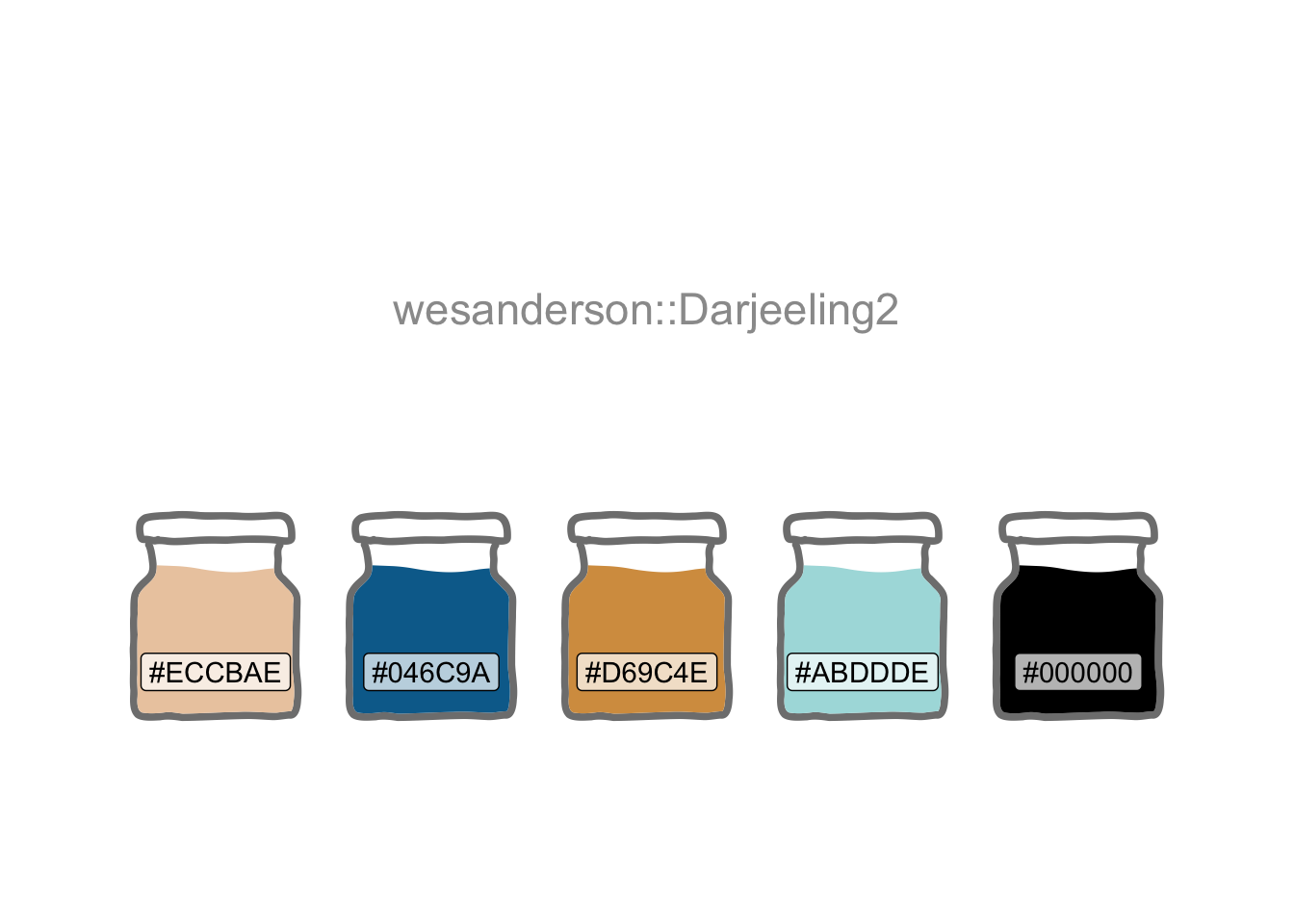``case_df <- readRDS("case.rds")``
``````url <-

file_name <- basename(url)

url |> basename

``geocodes <- read_csv("ukpostcodes.zip")``

The data need a bit of wrangling. And there is also the opportunity to try the newest column-wise enhancements to mutate: `mutate_if` and `mutate_at` have been superseded by `mutate` with `across`.

``````wide_df <- case_df |>
pivot_wider(names_from = X1, values_from = X2) |>
select(all_of(plan_colnames)) |>
mutate(
across(c(property_list, property_cons), \(vec) na_if(vec, "N/A")),
across(c(app_comp, decision), \(vec) na_if(vec, ""))
)

tidy_df <- wide_df |>
mutate(
dec_date = date_parse(dec_date, format = "%d %b %Y"),
dec_year = get_year(dec_date),
proposal_dev = str_to_lower(proposal_dev),
property_pcode = str_replace(property_pcode, "SW10(?!\\s)", "SW10 "),
app_comp = str_to_upper(app_comp) |>
str_remove_all("[:punct:]") |>
str_remove_all("\\b(?:AND|LTD|CO|LIMITED|UK|GROUP|LLP)\\b") |>
str_squish(),
decision = fct_explicit_na(decision, na_level = "Other"),
decision = str_replace(decision, "/", " / "),
dec_lump = fct_lump(decision, prop = 0.03),
basement = if_else(str_detect(proposal_dev, "basement"), "Yes", "No"),
property_listed = case_match(
property_list,
c("II", "II*", "2", "2*") ~ "Yes",
.default = "No"
),
app_comp = replace_na(app_comp, "None"),
property_cons = if_else(property_cons == "" | is.na(property_cons),
"None", property_cons
),
proposal_dev = if_else(proposal_dev == "" | is.na(proposal_dev),
"None", proposal_dev
),
across(where(is.character), str_trim),
across(c("app_comp", "proposal_type", "property_cons"), factor)
) |>
left_join(geocodes, by = join_by(property_pcode == postcode))

tidy_df |>
count(dec_lump) |>
arrange(desc(n)) |>
rename("Decision" = dec_lump, "Count" = n)``````
Decision Count
Grant Planning Permission / Consent 5336
Withdrawn by Applicant 1123
Other 847
Refuse Planning Permission / Consent 752
Discharge of Conditions - Grant 626
Raise No Objection 418

quanteda to look at key words in context (kwic).

I’d like to review planning applications by theme. So I’ll first need to get a sense of what the themes are by plotting the words which appear most frequently.

``````plus_words <-
c("new",
"pp",
"two",
"one",
"dated",
"withdrawn",
"flat",
"x",
"permission",
"rear",
"first",
"second",
"planning",
"floor",
"erection"
)

words <- tidy_df |>
corpus(text_field = "proposal_dev",
doc_vars = c("dec_date", "proposal_type",
"decision", "dec_year")) |>
dfm(
remove = c(stopwords("english"), plus_words),
remove_numbers = TRUE,
remove_punct = TRUE) |>
textstat_frequency() |>
mutate(feature = fct_reorder(feature, frequency))

words |>
ggplot(aes(feature, frequency)) +
geom_col(fill = cols) +
coord_flip() +
labs(x = NULL, y = NULL,
title = "Frequent Planning Proposal Words",
caption = "Source: RBKC Planning Search")``````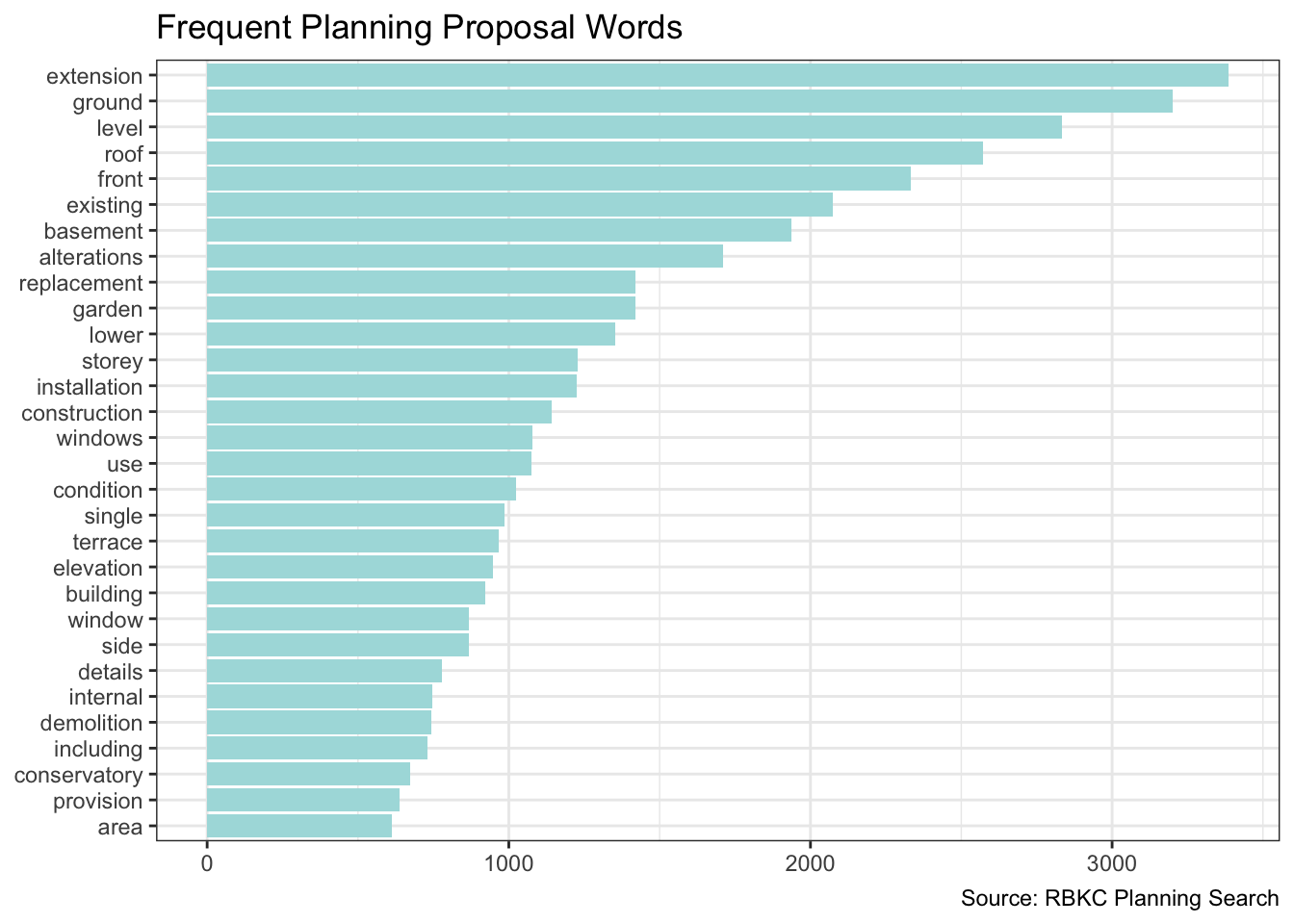Now I can create a theme feature.

``````remapped_df <- tidy_df |>
mutate(
theme = case_when(
str_detect(proposal_dev, "basem|excav") ~ "Basement or Excavation",
str_detect(proposal_dev, "xten|vatory|torey") ~ "Extension, Conservatory \nor Storey",
str_detect(proposal_dev, "windo|doo") ~ "Windows or Doors",
str_detect(proposal_dev, "roof") ~ "Roof",
str_detect(proposal_dev, "rrac|dsc|garde") |
str_detect(proposal_type, "Tree") ~ "Trees, Landscaping, \nGarden or Terrace",
.default = "Other"
),
outcome = case_when(
str_detect(decision, "Gran|No Ob|Accep|Lawf") ~ "Positive",
str_detect(decision, "Refus") ~ "Refuse",
str_detect(decision, "Withdr") ~ "Withdrawn",
.default = "Other"
)
)``````

I also want to compare house sales with planning applications over time. So, I’ll re-use the SPARQL query from House Sales.

``````tic()

endpoint <- "https://landregistry.data.gov.uk/landregistry/query"

query <- 'PREFIX  text: <http://jena.apache.org/text#>
PREFIX  ppd:  <http://landregistry.data.gov.uk/def/ppi/>
PREFIX  lrcommon: <http://landregistry.data.gov.uk/def/common/>

WHERE
_:b0 <http://www.w3.org/1999/02/22-rdf-syntax-ns#first> lrcommon:postcode .
_:b0 <http://www.w3.org/1999/02/22-rdf-syntax-ns#rest> _:b1 .
_:b1 <http://www.w3.org/1999/02/22-rdf-syntax-ns#first> "( SW10 )" .
_:b1 <http://www.w3.org/1999/02/22-rdf-syntax-ns#rest> _:b2 .
_:b2 <http://www.w3.org/1999/02/22-rdf-syntax-ns#first> 3000000 .
_:b2 <http://www.w3.org/1999/02/22-rdf-syntax-ns#rest> <http://www.w3.org/1999/02/22-rdf-syntax-ns#nil> .
?item ppd:hasTransaction ?ppd_hasTransaction .
?item ppd:pricePaid ?ppd_pricePaid .
?item ppd:transactionCategory ?ppd_transactionCategory .
?item ppd:transactionDate ?ppd_transactionDate .
?item ppd:transactionId ?ppd_transactionId

OPTIONAL { ?item ppd:estateType ?ppd_estateType }
OPTIONAL { ?item ppd:newBuild ?ppd_newBuild }
OPTIONAL { ?item ppd:propertyType ?ppd_propertyType }
OPTIONAL { ?item ppd:recordStatus ?ppd_recordStatus }
}'

sales <- SPARQL(endpoint, query)

toc()``````
``196.543 sec elapsed``

Let’s now bind the data into one tibble and summarise the transaction volumes over time.

``````sales_df <- sales\$results |>
as_tibble() |>
mutate(
date = as_datetime(ppd_transactionDate) |> as_date(),
dataset = "Sales"
) |>
summarise(volume = n(), .by = c(date, dataset))

app_df <- remapped_df |>
mutate(
date = dec_date,
dataset = "Planning"
) |>
summarise(volume = n(), .by = c(date, dataset))

compare_df <- bind_rows(app_df, sales_df)

summary_df <- compare_df |>
filter(date >= min(sales_df\$date)) |>
mutate(date = date_build(get_year(date), get_month(date), "last")) |>
summarise(volume = sum(volume), .by = c(date, dataset))``````

The visualisation below does suggest that home owners “start digging” when they can’t sell. At least in this part of London.

``````monthly_ts <- summary_df |>
mutate(date = yearmonth(date)) |>
as_tsibble(key = dataset, index = date)

monthly_ts |>
ggplot(aes(date, volume, colour = dataset)) +
geom_line(key_glyph = "timeseries") +
scale_colour_manual(values = cols[c(2, 3)]) +
labs(x = NULL, y = NULL, colour = NULL,
title = "Monthly Property Transaction Volume in SW10",
caption = "Sources: Land Registry & RBKC Planning"
)``````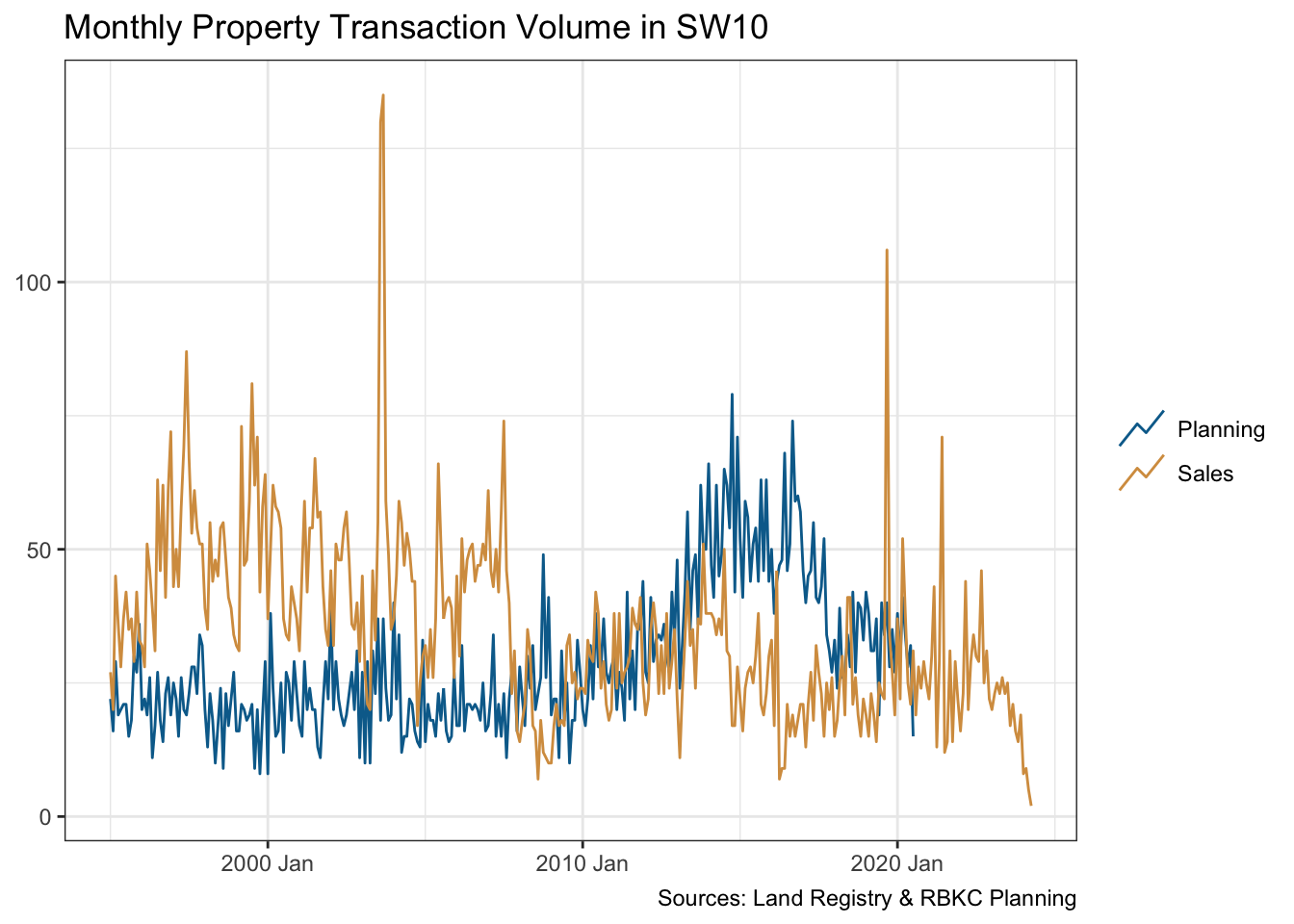Time-series data may have an underlying trend and a seasonality pattern. I’ll use the seasonal package to decompose each time-series. Each exhibit annual seasonality which evolves over time.

``````monthly_ts |>
model(stl = STL(volume ~ season())) |>
components() |>
autoplot() +
scale_colour_manual(values = cols[c(2, 3)]) +
labs(x = NULL, title = "Time Series Decomposition")``````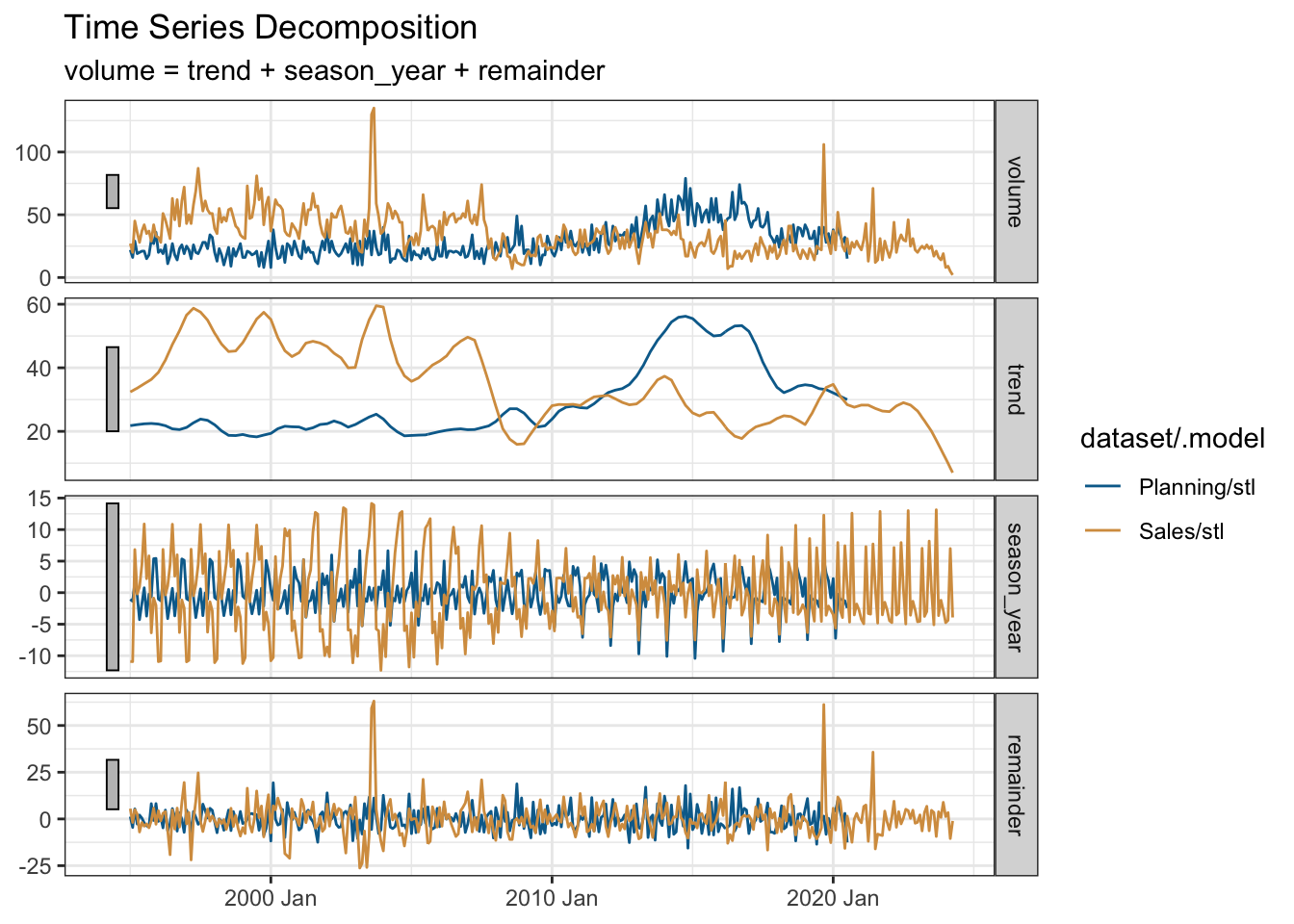We also see some inverse correlation between the two time-series re-affirming the visual conclusion that planning applications increase when the housing market is depressed.

``````monthly_ts |>
pivot_wider(names_from = dataset, values_from = volume) |>
CCF(Sales, Planning, lag_max = 6) |>
autoplot() +
labs(title = "Correlation Between Sales & Planning") +
theme(axis.text.x = element_text(angle = 45, hjust = 1))``````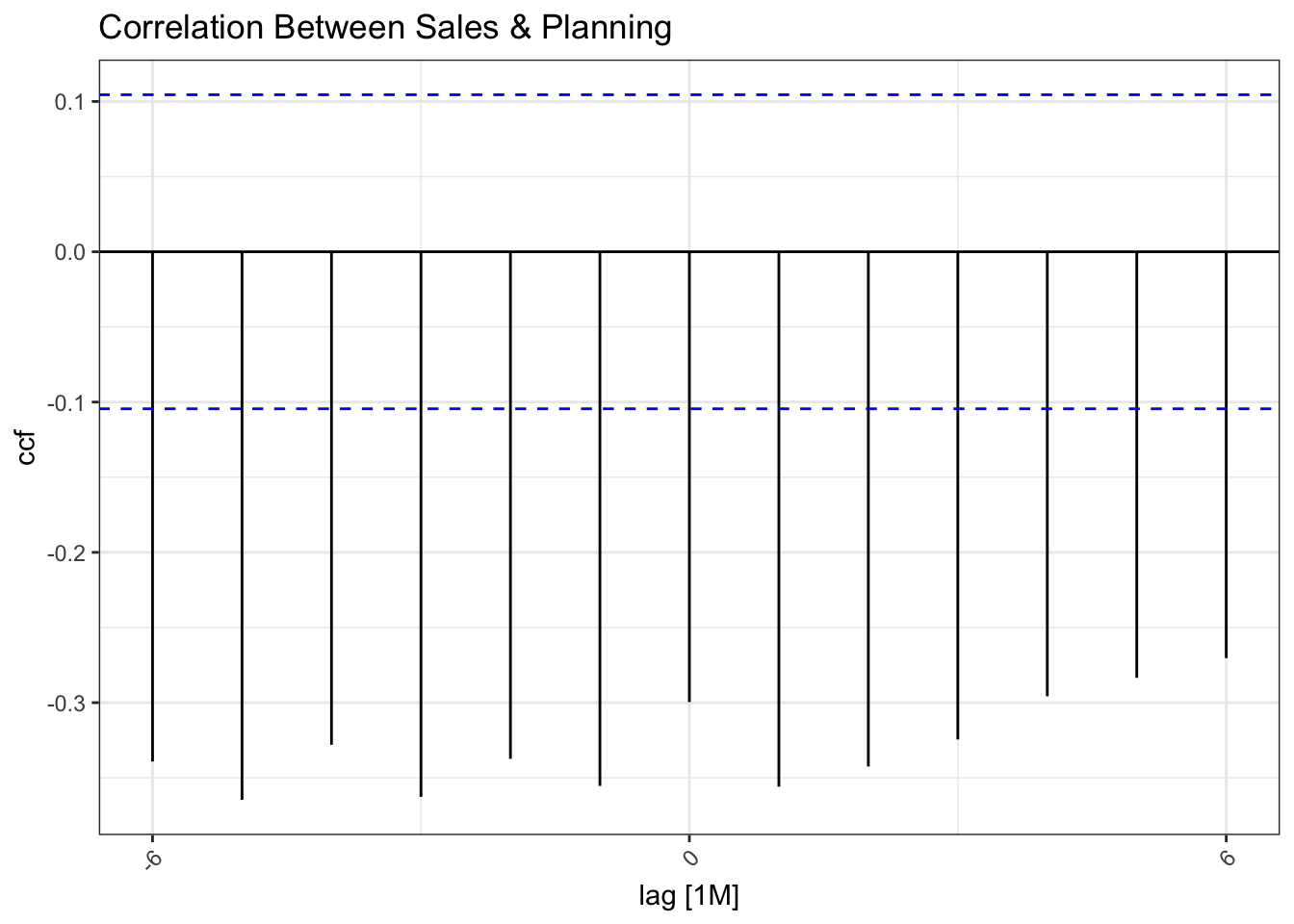The overall volumes of planning applications and house transactions in SW10 are fairly similar.

``````summary_df |>
summarise(total = sum(volume), .by = dataset) |>
rename("Dataset" = dataset, "Count" = total)``````
Dataset Count
Planning 8926
Sales 11764

Earlier, I added a “theme” feature to the data. So let’s take a look at the volume of applications over time faceted by theme and coloured by the outcome. We see that the rise in planning applications is fuelled by basements or excavations, and work on outside landscaping and terracing. So perhaps we do “dig” when we can’t sell.

``````remapped_df |>
ggplot(aes(dec_year, fill = outcome)) +
geom_bar() +
facet_wrap( ~ theme, nrow = 2) +
scale_fill_manual(values = cols[c(1:4)]) +
labs(
title = "Planning Application Themes",
x = NULL, y = NULL,
caption = "Source: RBKC Planning Search"
)``````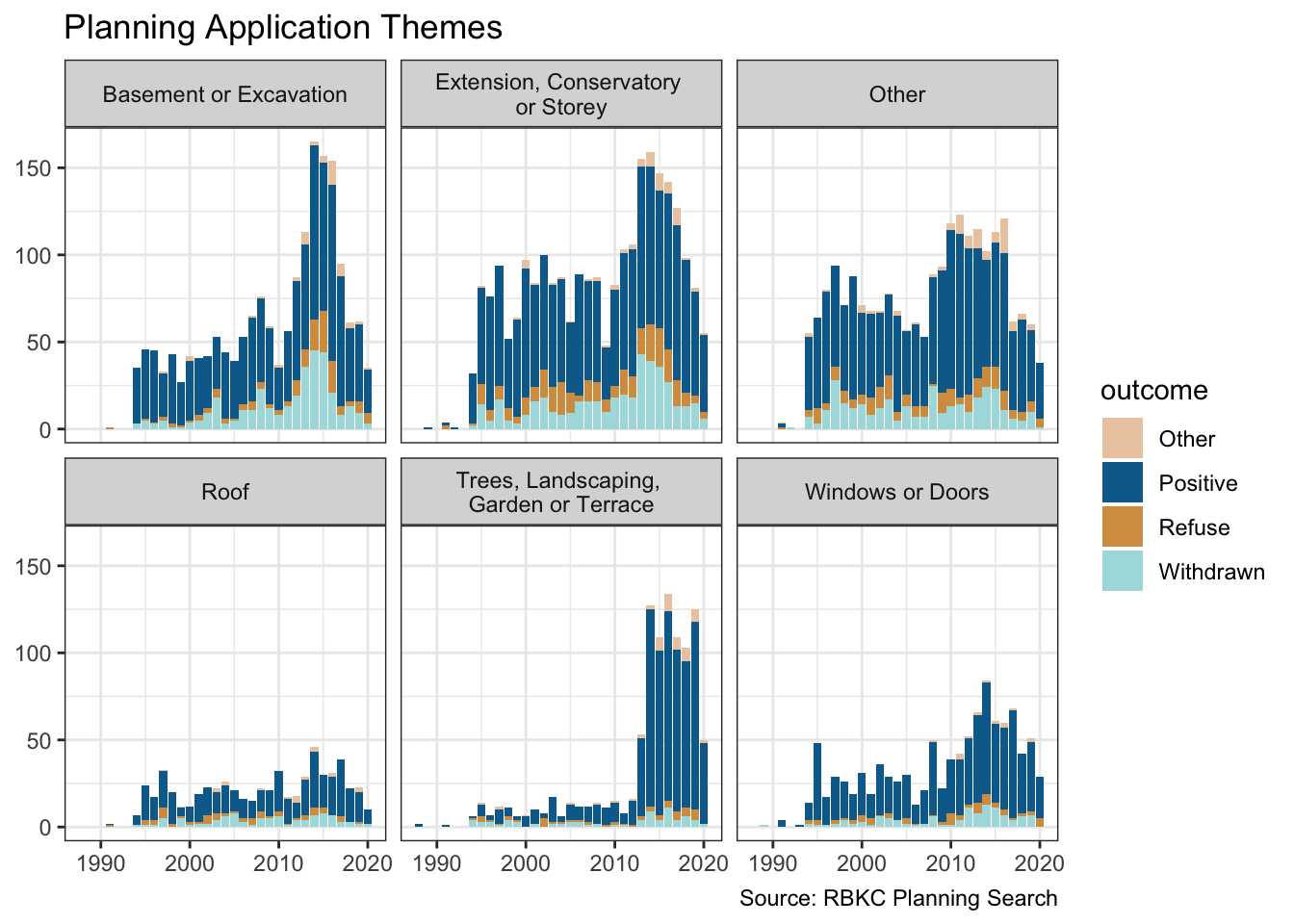## R Toolbox

Summarising below the packages and functions used in this post enables me to separately create a toolbox visualisation summarising the usage of packages and functions across all posts.

``used_here()``
Package Function
DT datatable
SPARQL SPARQL
base as.numeric, basename, c, is.na, library, min, readRDS, saveRDS, sum
clock date_build, date_parse, get_month, get_year
conflicted conflict_prefer, conflict_prefer_all, conflict_scout
dplyr across, arrange, bind_rows, case_match, case_when, count, desc, filter, if_else, join_by, left_join, mutate, n, na_if, rename, select, slice_head, summarise
fabletools components, model
feasts CCF, STL
forcats fct_explicit_na, fct_lump, fct_reorder
ggplot2 aes, autoplot, coord_flip, element_text, facet_wrap, geom_bar, geom_col, geom_line, ggplot, labs, scale_colour_manual, scale_fill_manual, theme, theme_bw, theme_set
htmlwidgets saveWidget
lubridate as_date, as_datetime
purrr list_rbind, map
quanteda corpus, dfm, kwic, phrase
quanteda.textstats textstat_frequency
rvest html_attr, html_element, html_elements, html_table, html_text
stopwords stopwords
stringr str_c, str_detect, str_extract, str_remove_all, str_replace, str_squish, str_to_lower, str_to_upper
tibble as_tibble, tibble
tictoc tic, toc
tidyr pivot_wider, replace_na
tidyselect all_of, where
tsibble as_tsibble, yearmonth
usedthese used_here